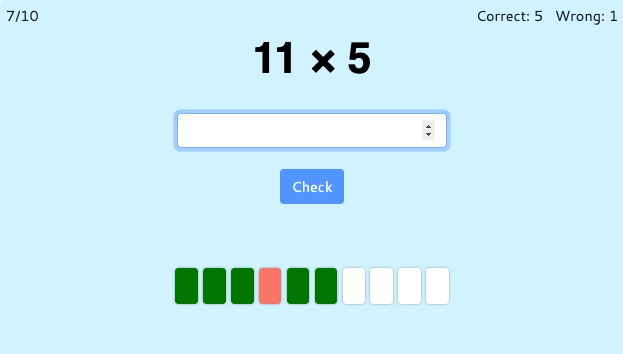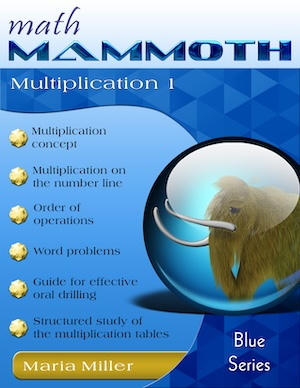MM Practice
×
Multiplication
Division
Place Value
Fractions & Decimals
Measurement
Statistics & Probability
Pre-Algebra
Money
Kindergarten
Geometry

# Multiplication TablesOnline practice

On this page, you can practice any combination of the multiplication tables — very helpful for students in elementary and middle school. You can practice any single times table (such as multiplication by 9), or several tables (such as tables of 2 and 5), or all of them.

You can choose timed or untimed practice, the number of practice problems, and which exact times tables you'd like to work on.

Screenshot:OR
Practice for a set time: min### Math Mammoth Multiplication 1

A self-teaching worktext that covers the concept of multiplication from various angles, word problems, a guide for structural drilling, and a complete study of all 12 multiplication tables.